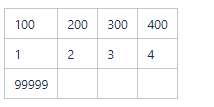# Groovy 17 – Multidimensional Array

Usually Multidimensional Arrays are made when you want to create a table.

In Oracle Sales Cloud, I have not yet saw a need for Multidimensional Array, but you can make them in groovy.

I wills how you how in the following examples.

This  is how a Multidimensional Array looks like:

def myArray = [[100,200,300,400],[1,2,3,4],];

So this means that the array , myArray , has 3 dimensions, which basically looks visually as 3 rows in a table.Now you can access the elements of the array by using loops.

The most common way is by using 2 for loops, the first loop will cycle through the dimensions and the second for loop will cycle through the items of each dimension

Now what I will do next is use a formula field to display the same tabel as above.

I will also use few things from the previous lessons, like sub-strings and string replace method.

So I made a formula field of type text and set tit to be multi-line.

Now the code I sue the following:

def myArray = [[100,200,300,400],[1,2,3,4],];
def  result  = []

def row_1 = myArray.size()
def row_2 = myArray.size() + row_1 +1

for(int row = 0 ; row<myArray.size();row++){

for(int column =0; column<myArray[row].size();column++){

}

}

def string_val = substringAfter(substringBefore(result.toString(),‘]’),‘[‘)
def newString = string_val .replaceAll(‘,’,)

return  newStringand the output of this is as belowNow lets explain the code above:

def myArray = [[100,200,300,400],[1,2,3,4],];

— this is my multidimensional array which has 3 dimensions

def  result  = []
— this is simple array in which I collect the out put of the for loops,
I use this because I want to split in rows the dimensions of the array

— otherwise if I return directly the string output of the for loops it will be display
everything on 1 single line, so it will defeat the
purpose of makings a table out of the multidimensional array.

def row_1 = myArray.size()
def row_2 = myArray.size() + row_1 +1
— here I calculate where the rows should be split

for(int row = 0 ; row<myArray.size();row++){
for(int column =0; column<myArray[row].size();column++){
}
}

— here is where we cycle throw the elements of the array.

— the first for loops cycles through the dimensions, so the variable row  will
take one by one the values of 0, 1 and 2

— the second for loop cycles through the items in each dimension,
so when row = 0 then  column takes one by one value of 0,1,2,3

–remember form the array lesson, the index of an arrays tarts at 0

— here i am using the simple array created early to collect each dimension
with its elements

— \t  – this is a string escape sequence that adds a tab white space between
each element so thy are not one next to each other, for visibility purpose

— because i want to display this as a table, I am adding a new line
(see the string lesson) where I previously calculated a new row should start

def string_val = substringAfter(substringBefore(result.toString(),‘]’),‘[‘)
— now I am removing the [ ] that come along when displaying an array
and also I am putting all the elements of the array in the new string string_val

def newString = string_val .replaceAll(‘,’,)
— here  anew string is made,newString  in which all the commas are removed
between the elements.
(remember form the String lesson, Strings are  immutable)

return  newString
-now I am displaying the result into the formula field
(see formula field lesson)

So as you saw I have used in this code notions of previous lessons,
and this is an example how all can come together .

How To Add Items in the Multidimensional Array.

From the start I can tell you you can not use add(), as multidimensional array is immutable.

You can do the following:

myArray = 1//- this ill introduce item 1 to dimension 1 on index 0

or

myArray.get(2).set(0777777);
//– this will basically replace value 99999 with
// 777777 in dimension 3 at index 0

Also This is a good opportunity to discuss java.lang.IndexOutOfBoundsException.

This JAVA error means that an non existing index from an array
is called in the code.

For example if you do this: myArray.get(2).set(1, 777777);
you will get  java.lang.IndexOutOfBoundsException

Because you are trying to set a value in dimension 3 at index 1.

Dimension 3 has only one index and that is 0,
there for the error pops up when code executes.

I hope you found this useful even if the example is quite silly,
but at least it gives you an idea of how Multidimensional  Arrays work in Oracle Sales Cloud.